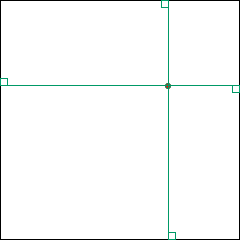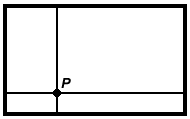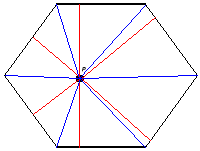#### You may also likeFour vehicles travelled on a road. What can you deduce from the times that they met?### A Scale for the Solar System

The Earth is further from the Sun than Venus, but how much further? Twice as far? Ten times?### Swimmers

Swimmers in opposite directions cross at 20m and at 30m from each end of a swimming pool. How long is the pool ?

# Pentagonal

##### Age 14 to 16Challenge Level

Laura sent us her work on this problem. Well done, Laura!Let the square have side length $l$. Then its perimeter is $4l$. Also, we can see from the diagram above that the sum of the distances from the point to the top and bottom sides is $l$, and similarly the sum of the distances from the point to the left and right sides is $l$, so the total sum of the distances is $2l=\frac{1}{2}\times 4l$, which is constant and half of the perimeter.Let the rectangle have height $h$ and width $w$. Then its perimeter is $2(h+w)$. This is very similar to the square. Here the sum of the distances from $P$ to the top and bottom sides is $h$, and the sum of the distances from $P$ to the right and left sides is $w$, so the total sum of the distances is $h+w=\frac{1}{2}\times 2(h+w)$ is constant, and again is half of the perimeter.This hexagon isn't regular, but it is equilateral (all its sides have the same length, $l$, say). I've drawn on the perpendicular lines (in red) from $P$ to the sides, and have also divided the hexagon into six triangles (in blue), each with base $l$ and height the distance from that side to the point. The area of the hexagon can therefore be written as $\frac{1}{2}(\textrm{sum of the heights})\times l$. Since this is constant, so is the sum of the heights.\par In the same way, the sum of the distances in an equilateral pentagon is constant.